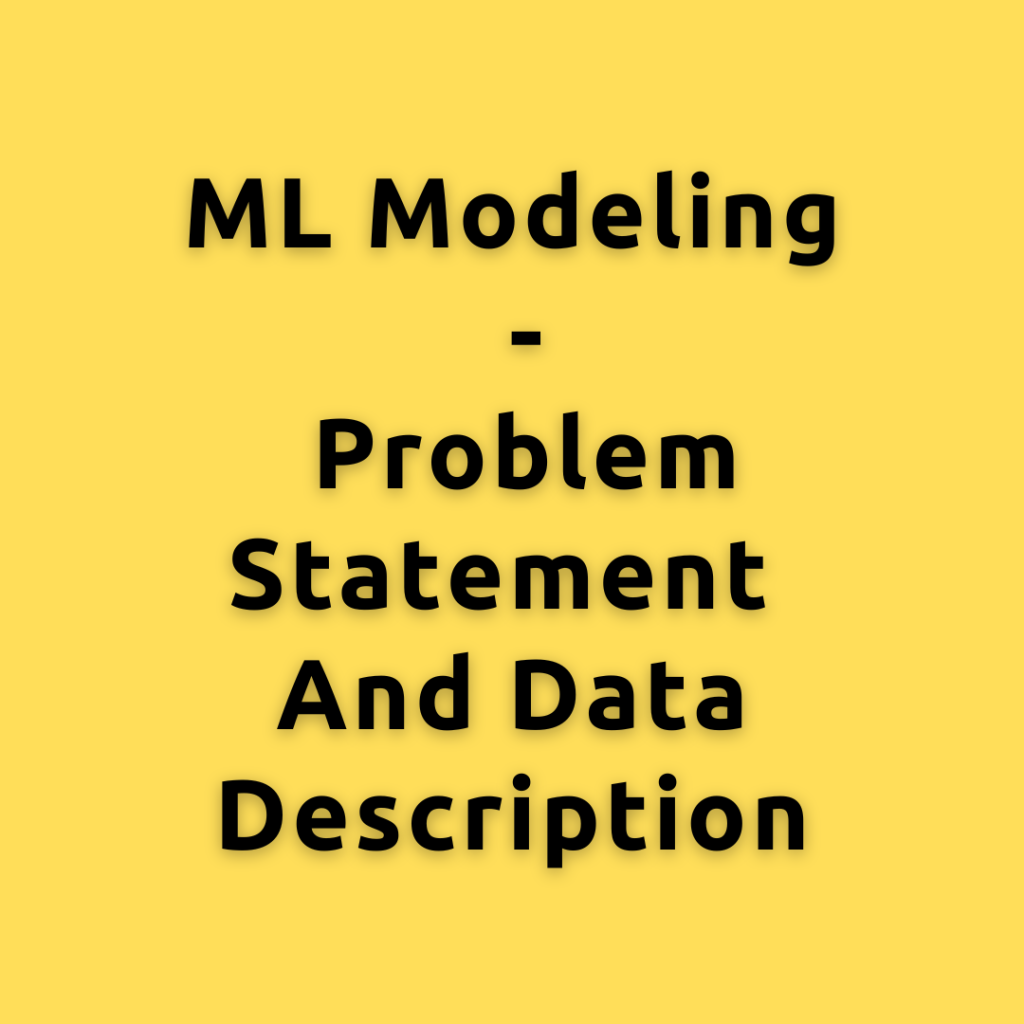## ML Modeling – Problem statement and Data description

ML modeling is the step where machine learning is used to find patterns in data and use that learned knowledge to predict an outcome. The type of ML modeling we are going to solve in this problem is called ‘Churn Modeling’. Let’s first understand the Churn modeling problem statement and then go over the data …